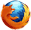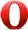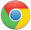# JavaScript random() 方法JavaScript Math 对象

Math.random();

## 定义和用法

random() 方法可返回介于 0（包含） ~ 1（不包含） 之间的一个随机数。

## 浏览器支持Math.random()

## 返回值

Number 0.0 ~ 1.0（不包含） 之间的一个伪随机数。

## 技术细节

 JavaScript 版本： 1

## 实例

Math.floor((Math.random()*10)+1);

## 实例

Math.floor((Math.random()*100)+1);

## 实例

function getRndInteger(min, max) { return Math.floor(Math.random() * (max - min) ) + min; }

## 实例

function getRndInteger(min, max) { return Math.floor(Math.random() * (max - min + 1) ) + min; }JavaScript Math 对象